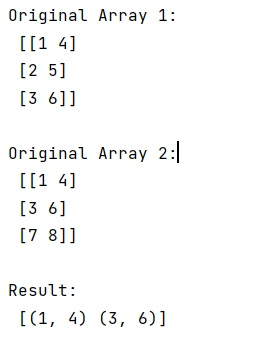# How to get intersecting rows across two 2D NumPy arrays?

Learn, how to get intersecting rows across two 2D NumPy arrays in Python?
Submitted by Pranit Sharma, on February 22, 2023

NumPy is an abbreviated form of Numerical Python. It is used for different types of scientific operations in python. Numpy is a vast library in python which is used for almost every kind of scientific or mathematical operation. It is itself an array which is a collection of various methods and functions for processing the arrays.

## Intersecting rows across two 2D NumPy arrays

Suppose that we are given two 2D numpy arrays and we need to get the intersecting (common) rows across two 2D numpy arrays.

For this purpose, we will use numpy.intersect1d() method which is used to find the intersection of two arrays. It returns the sorted, unique values that are in both of the input arrays.

Let us understand with the help of an example,

## Python code to get intersecting rows across two 2D NumPy arrays

```# Import numpy
import numpy as np

# Creating two numpy arrays
arr1 = np.array([[1,4],[2,5],[3,6]])
arr2 = np.array([[1,4],[3,6],[7,8]])

# Display original arrays
print("Original Array 1:\n",arr1,"\n")
print("Original Array 2:\n",arr2,"\n")

# getting shape for new array
rows, cols = arr1.shape

# Defining a data type
dt = {'names':['f{}'.format(i) for i in range(cols)],
'formats':cols * [arr1.dtype]}

# Finding intersection
res = np.intersect1d(arr1.view(dt), arr2.view(dt))

# Display result
print("Result:\n",res,"\n")
```

Output: